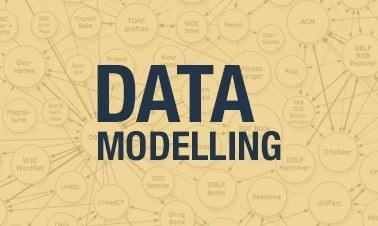# Modelling Data Distribution Assessment Test

10 Questions | Total Attempts: 105SettingsData distribution model is a statistical model that provides a set of assumptions pertaining the generation of some data and a larger population. Take this assessment test to evaluate your knowledge.

• 1.
A model is a simplification or approximation of reality and hence will not reflect all of reality.
• A.

True

• B.

False

• C.

Maybe

• D.

Depends on some factors

• 2.
Which of the following is a purpose for modeling?
• A.

Rastar

• B.

Creation

• C.

Prediction

• D.

Preferences

• 3.
An admissible model must be consistent with all the data points.
• A.

True

• B.

False

• C.

Not sure

• D.

None of the above

• 4.
The Poisson distribution is only meant for ____ integers.
• A.

Negative

• B.

Positive

• C.

Large

• D.

Small

• 5.
A statistical model is nonparametric if the parameter set ¶ is infinite dimensional.
• A.

True

• B.

False

• C.

Not defined

• D.

Depends on some factors

• 6.
Two statistical models are nested if the first model cannot be transformed into the second model...
• A.

True

• B.

False

• C.

Maybe

• D.

None of the above

• 7.
Which of the following set are nested distributions?
• A.

Vector

• B.

Mean

• C.

Base

• D.

Gaussian

• 8.
We can nest a model by...
• A.

Moving the parameters sideways

• B.

Moving the parameters forward

• C.

Moving the parameters backward

• D.

None of the above

• 9.
What can we get from a modeling data distribution?
• A.

Expected outcome

• B.

Fun outcome

• C.

Base angle

• D.

No outcome

• 10.
In a statistical model specified via mathematical equations, some of the variables do not have specific values, but instead have...
• A.

Outcome

• B.

Normal distribution

• C.

Probability distributions

• D.

Odd distribution

Related TopicsBack to top